The publishing house

MATFYZPRESS
of the
FACULTY OF MATHEMATICS AND PHYSICS
CHARLES UNIVERSITY, PRAGUE

has published the following lecture notes:FUNCTION SPACES LecturesSpring School in Analysis, Paseky 1999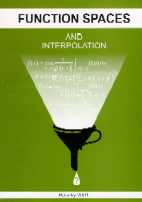FUNCTION SPACES AND INTERPOLATION LecturesSpring School in Analysis, Paseky 2001FUNCTION SPACES AND APPLICATIONS LecturesSpring School in Analysis, Paseky 2003FUNCTION SPACES AND APPLICATIONS LecturesSpring School in Analysis, Paseky 2005FUNCTION SPACES, INEQUALITIES AND INTERPOLATION LecturesSpring School in Analysis, Paseky 2007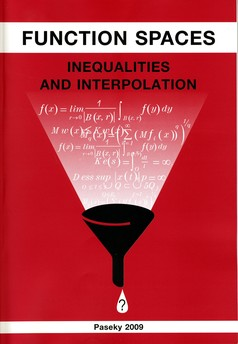FUNCTION SPACES, INEQUALITIES AND INTERPOLATION LecturesSpring School in Analysis, Paseky 2009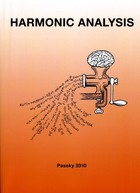HARMONIC ANALYSIS LecturesSpring School in Analysis, Paseky 2010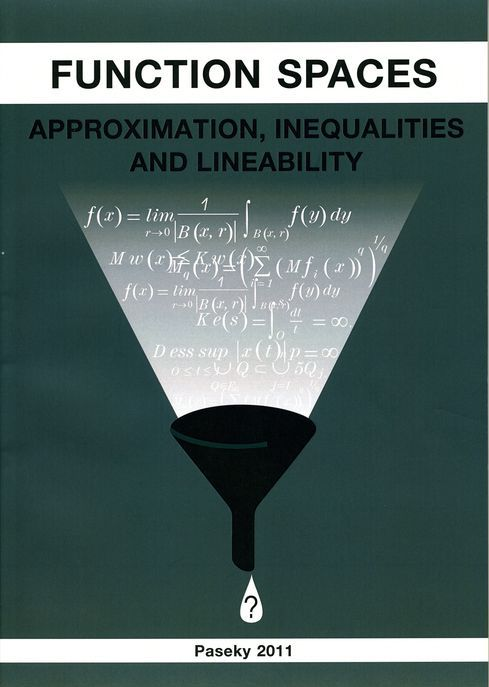FUNCTION SPACES, APPROXIMATION, INEQUALITIES AND LINEABILITY LecturesSpring School in Analysis, Paseky 2011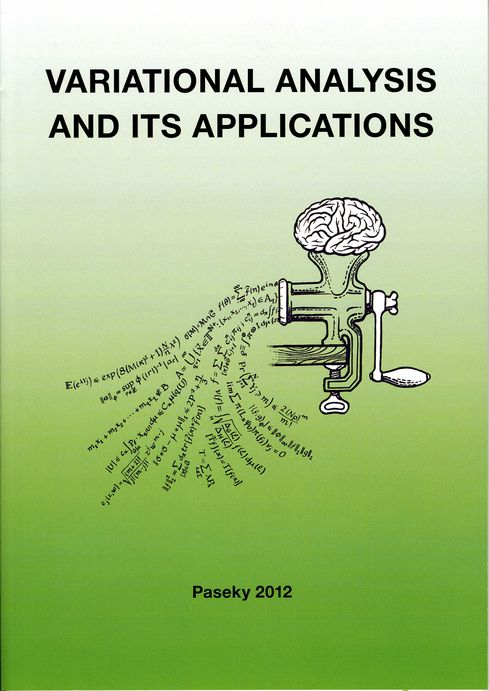VARIATIONAL AANALYSIS AND ITS APPLICATIONS LecturesSpring School in Analysis, Paseky 2012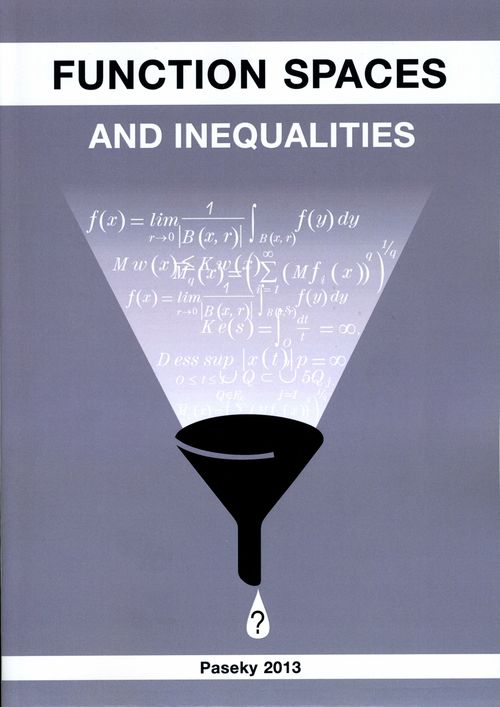FUNCTION SPACES AND INEQUALITIES LecturesSpring School in Analysis, Paseky 2013VARIATIONAL ANALYSIS AND ITS APPLICATIONS LecturesSpring School in Analysis, Paseky, April 2015FUNCTION SPACES AND LINEABILITY LecturesSpring School in Analysis, Paseky, June 2015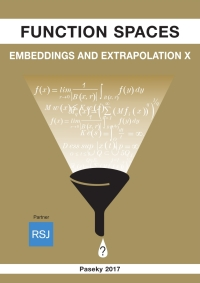EMBEDDINGS AND EXTRAPOLATION X LecturesSpring School in Analysis, Paseky, 2017MEASURE AND INTEGRAL byJaroslav Lukes, Jan MalyINTRODUCTION TO BANACH SPACES I,II byPetr Habala, Petr Hajek, Vaclav Zizler

• Lars Inge Hedberg and Yuri V. Netrusov: An axiomatic approach to function spaces and spectral synthesis
• Igor Verbitsky: Integral inequalities and function spaces associated with the Schroedinger operator

• W. Desmond Evans: Approximation numbers of Sobolev embeddings
• Pavel Schvartsman: The Whitney extension problem and Helly's theorem

• Grahame Bennett: The Classical Inequalities Revisited
• Björn Jawerth and Mario Milman: Lectures on Optimization, Image Processing and Interpolation Theory

• Anders Björn: Newtonian spaces: First-order Sobolev spaces on metric spaces
• Fernando Cobos: Interpolation theory and compactness
• Thierry Coulhon: Sobolev inequalities on non-compact Riemannian manifolds
• Peter A. HästE Muckenhoupt weights and variable-exponent function spaces

• Gerald B. Folland: The Many Faces of the Heisenberg Group
• Stéphane Jaffard: An introduction to Davenport series
• Thomas William Körner: Category and Probablistic Constructions in Harmonic Analysis

• Richard M. Aron: Linearity in non-linear problems
• Michael Lacey: Two Weight Inequalities in Harmonic Analysis: First Results and Outstanding Challenges
• Andrei K. Lerner: The local sharp maximal function and some applications

• Lars Diening: Lipschitz truncation, Discrete Sobolev Spaces
• Javier Duoandikoetxea: Forty years of Muckenhoupt weights
• Carlos Perez: Singular integrals and weights: a modern introduction
• Vladimir Stepanov: Integral operators on the semiaxis: boundedness, compactness and estimates of the characteristic numbers

• Marián Fabian: Separable Reductions and Rich Families in Theory of Fréchet Subdifferentials
• Alexander Ioffe: Metric Regularity. Theory and Applications
• David Russell Luke: Numerical Variational Analysis

• Pekka Koskela: Lectures on planar Sobolev extension domains
• Luboš Pick: Howto find the optimal partner
• Juan B. Seoane Sepúlveda: Lineability and Spaceability in Mathematics. Linearity in Nonlinear Settings

• Andrea Cianchi: Traces and embedding theorems in Sobolev type spaces
• David Cruz-Uribe: Extrapolation and Factorization
• Piotr Hajłasz: The Heisenberg groups

The introductory part, where the abstract theory of measure and integral is discussed, is followed by a chapter on integration in locally compact spaces. This culminates with the Riesz representation theorem. A brief introduction to measure theory on groups (Haar measure) is appended to this chapter. The study on integration on the real line contains the Lebesgue differentiation theorem and the Henstock-Kurzweil integral. From the topics on measure and integration on R^n, the covering theorems, differentiation of measures, density topology and approximately continuous functions are included. New proofs of the Rademacher and Besicovitch theorems are presented. A part of the book is devoted to the study of the theory of distributions, Fourier transform, approximation in function spaces and degree theory. The presentation on the line and surface integral is based on k-dimensional measure (possibly Hausdorff measure) and change of variables for Lipschitz surfaces. The chapter proceeds from an elementary approach (gradient, divergence, rotation) to more advanced one (differential forms on manifolds). The book is finished with integration of Banach space valued functions, where the Bochner, Pettis and Dunford integrals are discussed. Each chapter contains notes and remarks. An ample list of references is included at the end of the book. The text is intended for graduate and senior undergraduate students and young researchers.

CONTENT :

1. Measures and Measurable Functions
• The Lebesgue Measure
• Abstract Measures
• Measurable Functions
• Construction of Measures from Outer Measures
• Classes of Sets and Set Functions
• Signed and Complex Measures
2. The Abstract Lebesgue Integral
• Integration on R
• The Abstract Lebesgue Integral
• Integrals Depending on a Parameter
• The L^p Spaces
• Product Measures and the Fubini Theorem
• Sequences of Measurable Functions
• The Radon-Nikodym Theorem and the Lebesgue Decomposition
3. Radon Integral and Measure
• Radon Integral and Radon Outer Measure
• Radon Measures
• Riesz Representation Theorem
• Sequences of Measures
• Luzin's Theorem
• Measures on Topological Groups
4. Integration on R
• Integral and Differentiation
• Functions of Finite Variation and Absolutely Continuous Functions
• Theorems on Almost Everywhere Differentiation
• Indefinite Lebesgue Integral and Absolute Continuity
• Radon Measures on R and Distribution Functions
• Henstock-Kurzweil Integral
5. Integration on Rn
• Lebesgue Measure and Integral on Rn
• Covering Theorems
• Differentiation of Measures
• Lebesgue Density Theorem and Approximately Continuous Functions
• Lipschitz Functions
• Approximation Theorems
• Distributions
• Fourier Transform
6. Change of Variable Formula and k-dimensional Measures
• Change of Variable Theorem
• The Degree of a Mapping
• Hausdorff Measures
7. Surface and Curve Integrals
• Integral Calculus in Vector Analysis
• Integration of Differential Forms
• Integration on Manifolds
8. Vector Integration
• Measurable functions
• Vector Measures
• The Bochner Integral
• The Dunford and Pettis Integrals
9. Appendix on Topology
10. Bibliography and References
11. A Short Guide to the Notation
12. Subject Index

##### In TeX form available too.

The first chapters of this two volume text contain an up-to-date elementary and self-contained introduction to basic principles and techniques in Banach and Hilbert spaces, spectral theory of selfadjoint compact operators and fixed points. Besides the results that are usually presented in this area, the book contains a study on the James boundaries and the results of Asplund, Kadets, Lindenstrauss and Smulyan on the interplay of the differentiability of convex functions and geometry of Banach spaces. In the chapter on locally convex spaces, Banach-Dieudonne theorem, Eberlein-Smulyan theorem and Choquet representation theorem are included. The second part of the text leads the reader to several streams of contemporary research in Banach spaces and analysis on them. One chapter is devoted to the use of the Schauder basis in Banach spaces. Nonseparable Banach spaces are studied in the chapter on weak compact generating, where basic results on projectional resolutions of identity, Markusevic bases and various types of compacta are discussed. The chapter on superreflexive spaces contains Enflo's renorming theorem, the local reflexivity principle and the results of Kadets and Gurarii - Gurarii. The second volume of the text ends with a chapter on nonlinear analysis on Banach spaces. It contains the Aharoni, Heinrich, Kadets, Lindenstrauss and Mankiewicz results on homeomorphisms and Lipschitz homeomorphisms of Banach spaces, together with a brief discussion on the smooth variational principle, smooth approximation in Banach spaces and norm attaining operators. An important part of the book is the large number of exercises. They complement the results in chapters and are all accompanied with detailed hints for their solution. The text is intended for graduate and senior undergraduate students and young researchers.

CONTENT :

1. Preface
2. Basic Concepts in Banach Spaces
Banach spaces, subspaces, quotient spaces, Holder and Minkowski inequalities, classical spaces c_0, l_p, L_p[0,1], C[0,1], finite-dimensional subspaces, Hilbert spaces, Riesz' lemma, separability, orthonormal bases in Hilbert spaces. In Exercises: basic properties of Banach spaces, unconditional convergence.
3. Hahn-Banach Theorem, Dual Space
The Hahn-Banach theorem, dual space, dual operators, elementary Riesz' representation of duals to c_0, l_p, L_p[0,1] and C[0,1]. In Exercises: basic examples, duality, the Banach limit.
4. Weak Topologies, More on the Structure of Banach
Weak topology, weak star topology, second dual, bounded sets, Banach-Steinhaus theorems, Alaoglu's theorem, Goldstine's theorem, reflexivity, extreme points, the Krein-Milman theorem, James-Simons-Godefroy theorems on James boundaries of sets, James' characterization of weak compactness, the Eberlein-Smulyan and Krein-Smulyan theorems in separable spaces. In Exercises: basic properties of the weak and weak star topologies, extremal structure of convex sets, dual spaces, more on separation theorems.
5. Open Mapping Theorem, More on Classical Spaces
Banach's open mapping and closed graph theorems, projections and complementability, Auerbach bases, Sobczyk's theorem on complementability of c_0 in separable overspaces, Phillips' theorem on non-complementability of c_0 in l_infty, every separable Banach space is isometric to a subspace of C[0,1], every separable Banach space is isomorphic to a quotient of l_1, Schur's theorem on the coincidence of weak and norm convergence of sequences in l_1. In Exercises: subspaces, complementability, hyperplanes, projections, isomorphisms, isometries, operators, bilinear forms.
6. Differentiability of Norms and Duality
Gateaux and Frechet differentiability of norms, Smulyan's dual test, Kadets's renorming of spaces with separable dual by Frechet differentiable norms, the Asplund-Lindenstrauss result on Frechet differentiability on dense set of convex functions on spaces with separable dual, Mazur's theorem, Lindenstrauss' results on strongly exposed points of weakly compact sets. In Exercises: differentiability of convex functions and extremal structure of convex sets in classical spaces, rotundity, Kadets-Klee property, Fenchel duality, Mazur's intersection property.
7. Compact Operators on Banach Spaces
Finite rank and compact operators, spectrum and resolvent, self-adjoint operators on Hilbert spaces, spectral radius, spectral theory of compact self-adjoint operators on Hilbert spaces, normal operators, the Fredholm alternative, Fredholm operators, examples of integral operators. In Exercises: examples on spectra, Hilbert-Schmidt operators, Lomonosov's theorem on invariant subspaces.
8. Fixed Point Property
The Markov-Kakutani theorem, Banach's contraction principle, non-expansive mappings in Hilbert spaces, Schauder's theorems, applications to differential equations. In Exercises: examples of fixed points, Alspach's fixed-point-free isometry on a weakly compact set, Werner's proof of the Markov-Kakutani theorem.
9. Locally Convex Spaces
The notion of a locally convex space, metrizability, normability, finite dimensionality, separation, the bipolar theorem, the Mackey-Arens-Katetov theorem, the space of distributions, Choquet's representation theorem in metrizable case, the Banach-Dieudonne theorem, the Eberlein-Smulyan theorem, Kaplansky's theorem on countable tightness of the weak topology of a Banach space, the Banach-Stone theorem. In Exercises: examples, Sobolev spaces, the Grothendieck-Ptak results.
10. Schauder Bases
The notion of a Schauder basis, shrinking and boundedly complete bases, James' theorems on the characterization of reflexivity in terms of bases, Mazur's basic sequence theorem, the Krein-Milman-Rutman stability result, Pelczynski's theorems on subspaces of l_p spaces, unconditional Schauder bases, James' theorem on containment of l_1 and c_0, Pitt's theorem, James' space J, Khintchine's inequality, Rademacher functions in L_p. In Exercises: examples of Hamel and Schauder bases, isomorphisms, more on the James space.
11. Weakly Compact Sets and Spaces They Generate
Weakly compactly generated spaces, the notion of an Eberlein compact, the notion of a Markushevich's basis, Amir-Lindenstrauss' projectional resolutions of the identity on weakly compactly generated spaces, Davis-Figiel-Johnson-Pelczynski's factorization, Rosenthal's characterization of Eberlein compacta, weakly Lindelof spaces (Preiss-Talagrand), scattered compacts, the notion of a uniform Eberlein compact and renorming of C(K) by uniformly Gateaux differentiable norms (Argyros-Benyamini-Farmaki-Starbird-Troyanski), quasicomplements (Gurarii-Kadets), Polish or Czech complete balls in their weak topology (Godefroy, Edgar, Wheeler), absolutely summing operators, Pietsch's lemma and the Dvoretzky-Rogers theorem, the Dunford-Pettis property. In Exercises: examples, the three space property, weakly countably determined (Vasak) spaces, the Namioka property and other types of compacta.
12. Uniform Rotundity, Finite Representability
Uniform rotundity, the modulus of rotundity, uniform Frechet differentiability, duality, uniform convexity of L_p spaces for p in (1,infty), Kadets's result on unconditional bases in uniformly rotund spaces, the notion of finite representability, the local reflexivity principle, superreflexive spaces, Enflo's renorming of superreflexive spaces, the Gurarii-Gurarii-James theorem on Schauder bases in superreflexive spaces. In Exercises: examples on uniform convexity and smoothness, URED norms, the type and cotype of Banach spaces, Stegall's results on trees in dual spaces, the original definition of Tsirelson's space.
13. Application of Smoothness in Banach Spaces
Kadets's result that all infinite-dimensional separable reflexive Banach spaces are mutually homeomorphic, Aharoni's result that every separable Banach space is Lipschitz equivalent to a subset of c_0, the Heinrich-Mankiewicz method of linearization of Lipschitz maps, the smooth variational principle and the Bishop-Phelps theorem as its consequence, Lindenstrauss' result on the density of norm attaining operators, the Bonic-Frampton result on smooth approximation in separable spaces, subdifferentiability. In Exercises: examples on the smooth variational principle, rotundity, the Aharoni-Lindenstrauss space, the Gorelik principle, the Haar measure.
14. References
15. Index